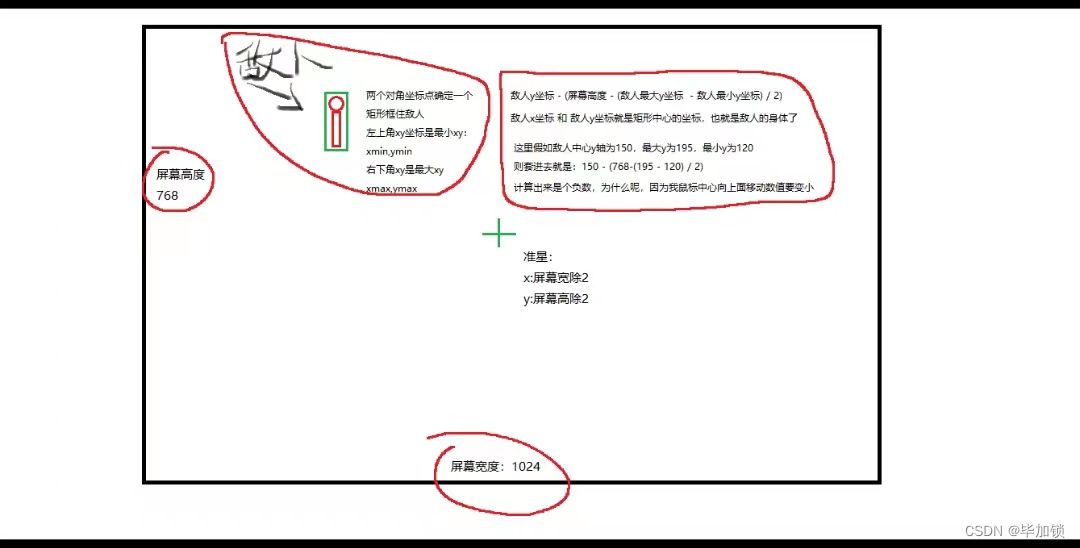# Ai实现FPS游戏自动瞄准 yolov5fps自瞄

1.xy坐标点与当前鼠标的xy坐标点距离计算

2.获取窗口句柄，本文使用的是根据窗口名称获取句柄

3.推理方式：本文使用的是GPU(为啥呢？速度快噻，因为我3060显卡噻~)。

4.屏幕宽高获取和敌人坐标获取和计算哪个是最近的敌人并控制鼠标去敌人身上和头上。（为什么说是身体和头部呢，因为还有一个方式，就是只需要敌人身体坐标就可以计算得出敌人头部坐标）干货噢~

5.还有鼠标按键状态获取。

``````
class Point():
def __init__(self, x1, y1, x2, y2):
self.x1 = x1
self.y1 = y1
self.x2 = x2
self.y2 = y2

class Line(Point):
def __init__(self, x1, y1, x2, y2):
super().__init__(x1, y1, x2, y2)

def getlen(self):
changdu = math.sqrt(math.pow((self.x1 - self.x2), 2) + math.pow((self.y1 - self.y2), 2))
return changdu``````

``````L1 = Line(x1, y1, x2, y2)  #传入两个xy坐标
L1.getlen() #return出两个坐标点的直线距离``````

``````# 这里是导入依赖，需要这些库
import math
import sys
import time

import torch
import win32api
import win32con
import win32gui
from PyQt5.QtWidgets import QApplication
from pynput.mouse import Controller
import mouse

#这里这俩class就是文章上面说的那个传入两个坐标点，计算直线距离的
class Point():
def __init__(self, x1, y1, x2, y2):
self.x1 = x1
self.y1 = y1
self.x2 = x2
self.y2 = y2
class Line(Point):
def __init__(self, x1, y1, x2, y2):
super().__init__(x1, y1, x2, y2)

def getlen(self):
changdu = math.sqrt(math.pow((self.x1 - self.x2), 2) + math.pow((self.y1 - self.y2), 2))
return changdu

#第一步：我们获取到某FPS游戏的窗口句柄
hwnd = win32gui.FindWindow(None, "穿越火线")
#这个方法是获取上面句柄窗口的窗口截图，用的是PyQt截图，有速度更快更好的方式的话可以换上
#截图完毕后保存在根目录的cfbg.bmp文件
def screen_record():
app = QApplication(sys.argv)
screen = QApplication.primaryScreen()
img = screen.grabWindow(hwnd).toImage()
img.save("cfbg.bmp")

#这里就是调用我们那yolo模型来进行推理啦，我设置的是cuda，也就是英伟达的GPU，因为cpu太慢了。
#如果自己的不能使用GPU推理的话把下面这两行改改，改成cpu的就可以了。
device = torch.device("cuda")
# 这里是定义屏幕宽高[其实这俩就是游戏所对应的分辨率，比如：游戏里1920*1080这里就是1920*1080]
game_width = 1024
game_height = 768
# 这边就是开始实时进行游戏窗口推理了
#无限循环 -> 截取屏幕 -> 推理模型获取到每个敌人坐标 -> 计算每个敌人中心坐标 -> 挑选距离准星最近的敌人 -> 如果左键是按下状态则控制鼠标移动到敌人的身体或者头部(本文计算方式是移动到头部)
while True:
# 截取屏幕
screen_record()
# 使用模型
model = model.to(device)
img = 'cfbg.bmp'
# 开始推理
results = model(img)
# 过滤模型
xmins = results.pandas().xyxy['xmin']
ymins = results.pandas().xyxy['ymin']
xmaxs = results.pandas().xyxy['xmax']
ymaxs = results.pandas().xyxy['ymax']
class_list = results.pandas().xyxy['class']
confidences = results.pandas().xyxy['confidence']
newlist = []
for xmin, ymin, xmax, ymax, classitem, conf in zip(xmins, ymins, xmaxs, ymaxs, class_list, confidences):
if classitem == 0 and conf > 0.5:
newlist.append([int(xmin), int(ymin), int(xmax), int(ymax), conf])
# 循环遍历每个敌人的坐标信息传入距离计算方法获取每个敌人距离鼠标的距离
if len(newlist) > 0:
# 存放距离数据
cdList = []
xyList = []
for listItem in newlist:
# 当前遍历的人物中心坐标
xindex = int(listItem - (listItem - listItem) / 2)
yindex = int(listItem - (listItem - listItem) / 2)
mouseModal = Controller()
x, y = mouseModal.position
L1 = Line(x, y, xindex, yindex)
# 获取到距离并且存放在cdList集合中
cdList.append(int(L1.getlen()))
xyList.append([xindex, yindex, listItem, listItem, listItem, listItem])
# 这里就得到了距离最近的敌人位置了
minCD = min(cdList)
# 如果敌人距离鼠标坐标小于150则自动进行瞄准，这里可以改大改小，小的话跟枪会显得自然些
if minCD < 150:
for cdItem, xyItem in zip(cdList, xyList):
if cdItem == minCD:
# 锁头算法：使用win32api获取左键按下状态，如果按下则开始自动跟枪
if win32api.GetAsyncKeyState(0x01):
# 控制鼠标移动到某个点：看不懂计算方式的话看文章下面讲解吧O(∩_∩)O
win32api.mouse_event(win32con.MOUSEEVENTF_MOVE, int(xyItem - game_width // 2),int(xyItem - (game_height - (xyItem - xyItem)) // 2), 0, 0)
break``````

win32api.mouse_event(鼠标行为：这里用的是MOVE相对移动, x坐标,y坐标, 0, 0)